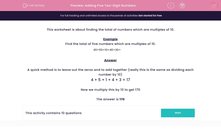### Comprehensive & curriculum aligned

In this worksheet, students add together five two-digit numbers. The numbers are all multiples of 10.This content is premium and exclusive to EdPlace subscribers.Key stage:  KS 2

Curriculum topic:   Maths and Numerical Reasoning

Curriculum subtopic:   Mixed Problems

Difficulty level:#### Worksheet Overview

This worksheet is about finding the total of numbers which are multiples of 10.

Example

Find the total of five numbers which are multiples of 10.

40 + 50 + 10 + 40 + 30 =

A quick method is to leave out the zeros and to add together (really this is the same as dividing each number by 10)

4 + 5 + 1 + 4 + 3 = 17

Now we multiply this by 10 to get 170